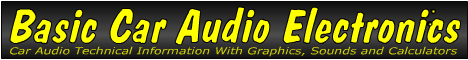Email   Home Page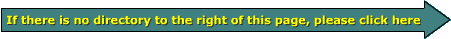x

 Web www.bcae1.com www.bcot1.com
Use F11 to go to full screen
Translate.

 Series vs Parallel There are 2 ways to connect multiple devices to a power source (e.g. speakers to an amplifier), series and parallel. Well... OK, there's also series/parallel. But we'll cover that on an upcoming page.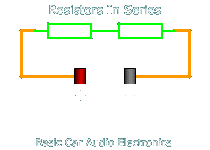Speakers in Series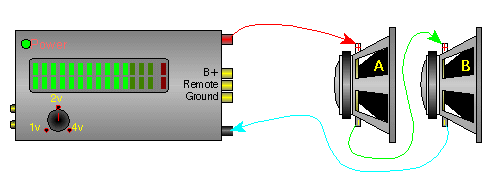In a series circuit (like the two above), the current must flow through one device to get to the next device. This means that the rate of current flow through all devices is the same. The voltage across each device depends on its impedance/resistance of each device and the current flowing through the circuit. When adding more components in a series circuit, the current flow decreases, if the applied voltage remains constant.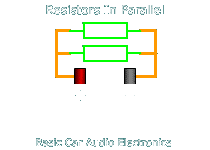Speakers in ParallelIn a parallel circuit (like the two examples above), each device is directly connected to the power source. This means that each device receives the same voltage. The amount of current flowing through each device is dependent on the impedance/resistance of that particular device. If devices are added to the power source in a parallel configuration, the current demand/flow from the power source increases. In the 2 diagrams below, you can see the relationship between the current flow out of the amplifier and the number of speakers. You can see that four speakers draws twice as much current from the amplifier than the two speaker configuration.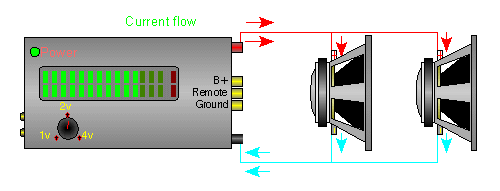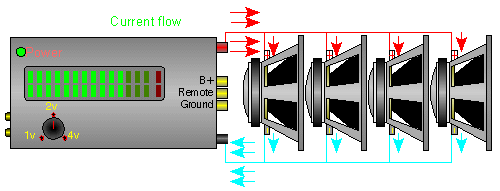When making any connections to any power source you must know the limits of the source, to prevent damage to the source. This means that if you connect too many speakers, in a parallel wiring configuration, to an amplifier (the power source in this case) it may well be damaged beyond repair. I've seen it happen, especially when the amplifier was improperly fused. For those Who Want to do the Math... In the diagrams/text above, we had either series OR parallel circuits. This section will show you what happens to the voltage, current and power dissipation in a series/parallel circuit. As was said before, in a series circuit, the 'current' in each device is the same. In a parallel circuit, the 'voltage' is the same across each device. In the following circuit, you can see that there are two 1000 ohm resistors in series with a single 400 ohm resistor. We know that the voltage across the two 1000 ohm resistors is going to be the same (because they're connected in parallel). We also know that the total current flow through the two parallel connected resistors will equal the current flow through the 400 ohm resistor.To calculate the total current, we should first simplify the circuit. This means we need to find the total resistance of the parallel network. For a simple circuit with two equal value resistors, we can simply divide the resistance of a single component by the total number of components. For this parallel network, we have two 1000 ohm resistors. Total resistance of parallel resistors = resistance of a single component/number of resistors Total resistance of parallel resistors = 1000/2 Total resistance of parallel resistors = 500 ohms If we had 3 parallel resistors, we'd divide 1000 by 3 to find the total resistance of the parallel resistors. Total resistance of parallel resistors = 1000/3 Total resistance of parallel resistors = 333.33.... ohms Now that we know that the parallel resistors are equal to a single 500 ohm resistor. Now that we have, esentially, one 500 ohm resistor in series with a 400 ohm resistor, we can calculate the total current through the circuit. We know that we have a 12 volt power source. We also know that the 500 ohm resistor in series with the 400 ohm resistor is equal to a 900 ohm resistor. Current flow through circuit = voltage across circuit/total circuit resistance Current flow through circuit = 12/900 Current flow through circuit = 0.0133 amps Now we can find the voltage across the individual components. To reduce the possibility of getting confused, calculate the voltage across the single resistor first. Voltage across the resistor = resistor's resistance*current flow through resistor Voltage across the resistor = 400*0.0133 Voltage across the resistor = 5.333 volts In a series circuit, all of the voltages across all of the individual series components will equal the power supply voltage. If we have a 12 volt source and the voltage across the 400 ohm resistor is 5.333 volts, we know that the voltage across the parallel pair of resistors is going to be 6.67 volts (12-5.333=6.67). To calculate the current through the parallel components... Current through a single 1000 ohm resistor = voltage across resistor/resistance Current through a single 1000 ohm resistor = 6.67/1000 Current through a single 1000 ohm resistor = .0067 amps Now that we know the voltage across each of the components and the current flow through each of the components, we can calculate the power dissipation for each component. Actually we could have done it as soon as we knew the voltage across the components but I decided to take the 'scenic' route. Power dissipation in the 400 ohm resistor = voltage across component*current through component Power dissipation in the 400 ohm resistor = 5.33*0.013 Power dissipation in the 400 ohm resistor = 0.071 watts Power dissipation in each 1000 ohm resistor = voltage across component*current through component Power dissipation in each 1000 ohm resistor = 6.67*.0067 Power dissipation in each 1000 ohm resistor = .045 watts This example used resistors but the same calculations would work for any resistive device. Although speakers are not purely resistive when being driven with AC voltage, the calculations here could be used to make rough calculations for voltage, current and power dissipation in speakers.

And a calculator to check your math if you want to try the above circuit with different values.

 Input Power Supply Voltage X? Volts Power Supply Voltage Y? Volts Resistor A Value? Ohms Resistor B Value? Ohms Resistor C Value? Ohms Output Total Power Supply Voltage Volts Total Power Supply Current Amps Total Resistance a||b Ohms Total Resistance a||b+c Ohms

 Voltage Resistor A Volts Current Resistor A Amps Power Dissipation Resistor A Watts Voltage Resistor C Volts Current Resistor C Amps Power Dissipation Resistor C Watts Voltage Resistor B Volts Current Resistor B Amps Power Dissipation Resistor B Watts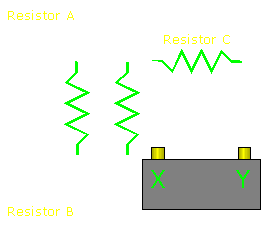You May Be Interested in My Other Sites
• This site was started for pages/information that didn't fit well on my other sites. It includes topics from backing up computer files to small engine repair to 3D graphics software to basic information on diabetes.
• This site introduces you to macro photography. Macro photography is nothing more than the photography of small objects. It can take quite a while to understand the limitations associated with this type of photography. Without help, people will struggle to get good images. Understanding what's possible and what's not possible makes the task much easier. If you need to photograph relatively small objects (6" in height/width down to a few thousandths of an inch), this site will help.
• If you're interested in air rifles, this site will introduce you to the types of rifles available and many of the things you'll need to know to shoot accurately. It also touches on field target competition. There are links to some of the better sites and forums as well as a collection of interactive demos.
• This site helps anyone new to computers and anyone with a basic understanding of computers with a desire to learn more about the internal components of a computer. If you have a computer that you'd like to upgrade but don't know where to start, this is a good site for you.
• This site is for those who want to begin racing karts but don't fully understand how the various parts work. It's mostly interactive demos that show how the various parts of the kart work.

 You should remember: 1.In a series circuit, the same current flows through all of the series connected components. 2.In a parallel circuit, the same voltage is applied to all of the parallel connected components.

```

```